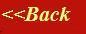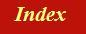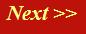#### Tutorials:

TEM alignment

STEM alignment

Wave interference

#### Research:

Diffractive imaginging

## The Fourier Transform:

The Fraunhofer diffraction pattern (as opposed to the Fresnel diffraction pattern) is mathematically identical to the Fourier transform, at least within certain approximations. We can therefore use our phase thread analogy to derive a pictorial representation of the Fourier transform. Some non- physicists are scared witless by the words ‘Fourier transform’, which is used everywhere in the field of wave optics. Unfortunately, it is really very hard to understand electron microscopy without understanding the Fourier transform.

On this page I hope to show that is easy to get a picture in your mind’s eye of what’s happening: learning to do Fourier transforms in your head is quite easy. When we talked about our Young’s slits experiment, we actually did a Fourier transform without realising it. Remember, in order to calculate how two waves would interfere with one another, we used a diagram like this: .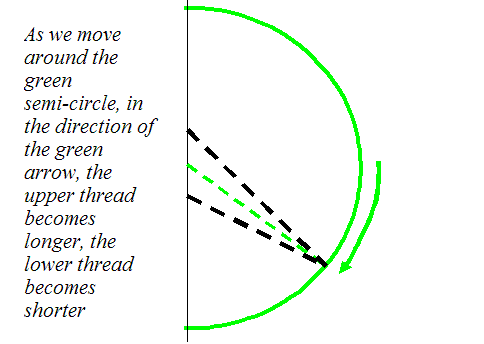If we wanted to represent our two slits mathematically, we would draw a function like this: .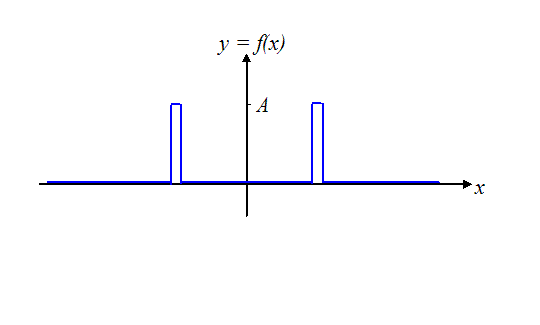The function (in blue) is zero everywhere except at the positions of the two slits where it has a value A (imagine the spikes are very narrow): we can think of this as the amplitude of the wave emanating from the slit. (In general, the value of A can be complex, but let’s assume it is real for the time being). I have been careful to draw the slits equal distances either side of the origin on the x-axis: this way the Fourier transform is a purely real function. If you remember, with two slits, a purely real function arrived over the green curved surface in the far-field (Fraunhofer) diffraction plane, and it looked like this: .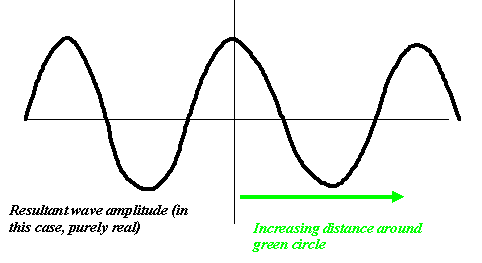This wavy line function is the Fourier transform of our original ‘two-spike’ function, shown above. Simple! .

Before we go any further, lets just think what would happen if we only had one slit, and it was positioned off- axis, like this: .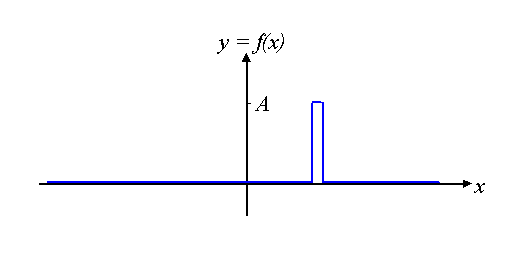Well, remember how we constructed our Young’s slits amplitude? If we only have one slit, only one phase thread arrives in the far-field. Relative to the green Gaussian surface we drew, a surface of constant phase of the wave emanating from the single slit will look like the blue line in the figure below: .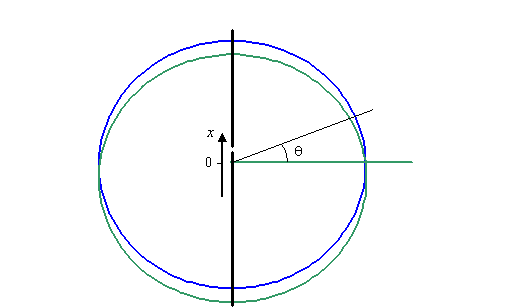In the central region of the Fraunhofer diffraction pattern, this blue line deviates from the green line as a linear function of the scattering angle, θ. So if we drew a graph of the path length difference between the green and the blue line in the region of small θ, the graph would look like this: .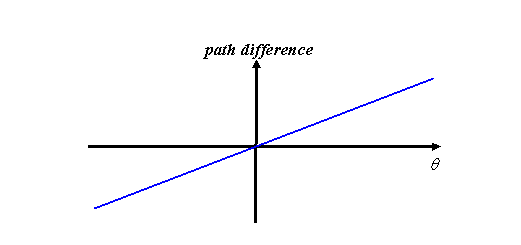Now what does the complex value of the wave from this slit look like? Well, there is only one thread contributing to its value, and this is simply increasing in phase constantly – in other words, it is itself a corkscrew function. Let’s plot the real part of this corkscrew as a solid line, and the imaginary part as a dotted line. As a function of θ, the angle around the green circle, the Fourier transform of a single (displaced) slit therefore looks like this: .If we were to measure the intensity of this wave distribution (which is all that we can do experimentally) then it is a rather boring function which just has a constant value, like this: .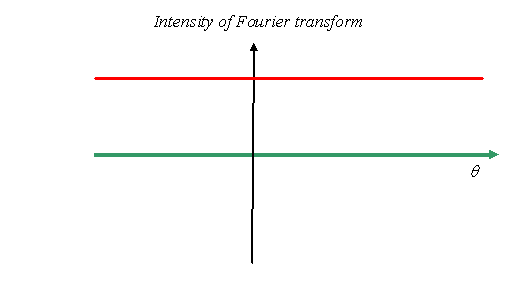Basically, because we only have one phase thread involved, there is no other wave to interfere with it, so all we are seeing is its constant amplitude (the length of its complex vector) squared. However, the important point I want to make here is that the underlying phase (i.e. the complex value of the amplitude) of the wave derived from an off-axis slit has a lot of structure in it: it is a complex function. The Fourier transform of a displaced spike is a corkscrew function. The more we displace the spike (i.e. the slit), then across the green Gaussian surface, this corkscrew has a higher and higher frequency (as a function of θ, the phase of the thread rotates more and more quickly).

This complex property of a Fourier transform is a central cause of confusion amongst many students. People are often taught that the Fourier transform of a spike (called mathematically a delta function) is a function of constant value. That’s true if the slit is on the axis of the calculation (that is, positioned at the centre of the green circle), but not if it is shifted. This phase structure matters a great deal, say if we consider how a lens would interact with the Fraunhofer diffracted amplitude.

Okay, so what happens when we have a general function, not just a spike or single slit? Well, there’re two separate questions here. One is ‘how do we mathematically define the Fourier transform?’ and the other is ‘what is the scattered amplitude in the Fraunhofer diffraction plane?’ Optics books will argue that the complex value of a Fraunhofer (note: not its intensity) is the Fourier transform of a wave being scattered from an object. This is not very precise. Two further questions arise. One is what it is we are taking the Fourier transform of (is it the scattering object or the wave that comes out of the scattering object), and the other is the geometric approximation in which it is true that the Fourier transform equals the Fraunhofer diffraction pattern. In most electron microscopy textbooks, these issues get mixed up. People talk of the Fourier transform of the atomic potential and/or the Fourier transform of an exit wavefield. The Ewald sphere takes into account the geometric approximation in the first case, the latter is used in the construction of the Abbe theory of imaging (e.g. for calculating the bright- field image or the contrast transfer function of the objective lens).

Let’s tackle the geometric approximation first. The Fraunhofer diffraction pattern exists, as we said before, when all the phase threads can be thought of as being parallel to one another. For a very large Gaussian surface (the green line in the first diagram), and a relatively compact source of waves, this condition is satisfied. However, for a single slit displaced by a distance D from the centre of the Gaussian surface, the path difference, p, as a function of θ around the green circle is given by p = D sin θ. Clearly, when θ= 90 degrees, the path difference for the wave emanating from the displaced slit is just exactly D: it can never get larger than this. However, the mathematical definition of a Fourier transform relies of the path difference p being exactly proportional to θ (the coordinate of the Fraunhofer diffraction plane). This is only true at small values of θ, when sin θ is approximately equal to θ (when θ is measured in radians). This small angle approximation is always assumed as true in any electron imaging situation (but not for Bragg diffraction at high angles – we will discuss this later).

For the time being, we won’t worry about the relationship between a scattering object and the wave it produces, we’ll just worry about how some general wave (usually over a two-dimensional surface) develops into a Fraunhofer diffraction pattern, a very long way from its source (and under the small scattering angle approximation).

So, remember our harbour wall. Lets now suppose that the wave disturbance within the harbour wall is not the same everywhere between the walls (i.e. we cannot represent it by an incident plane wave) but it has some complex value: complex in both senses of the word – a complicated structure in both modulus and phase. We can draw such a function as before, using a solid line to represent its real value and a dotted line to represent its imaginary value. Here’s an example function, which I will call f(x), plotted as a function of x, the distance across the mouth of the harbour wall.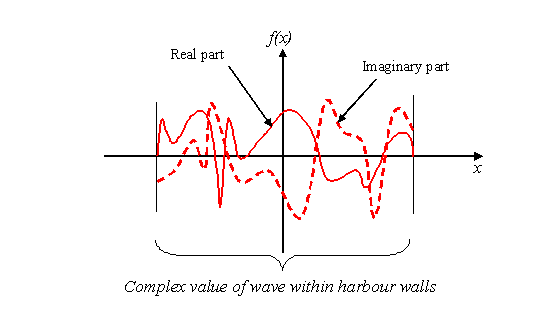We now attach a phase thread to every part of this function. As with our Young’s slits experiment, each thread will be allowed to propagate to a far off (green) Gaussian surface. We will colour the thread coming from the centre of the harbour wall as green: the phase of this thread is constant over the entire Gaussian surface. The only important difference between this experiment and Young’s slits (apart from the very large number of phase threads, or slits, that make up the total wave) is that each phase thread has the modulus and phase (i.e. the complex value) of the original wave (between the harbour walls) at the point where it is attached to the wave. So, the phase threads coming from the three points marked on our example wave function –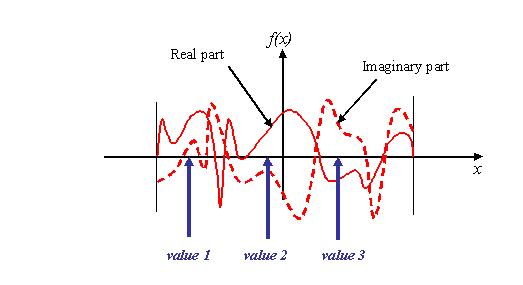have the following respective complex values: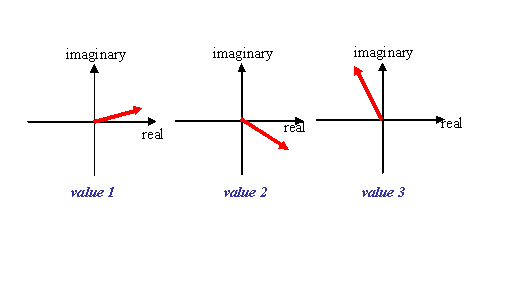Remember, these are just the vector plots of the real and imaginary parts of the value of the electron wave.

Now, at θ = 0, all the phase threads meet up on the green surface. Because all the phase threads are parallel (because the radius of the green circle is so large), their total lengths are all identical, so when we add them all together (because they all interfere with one another) we just have to add together every real and imaginary part of our original wave. That is, we integrate (add up) every complex value of f(x). We write this mathematically as: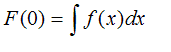Where F(0) is the Fourier transform of f(x) at a value of θ =0. So that’s easy. The value of a Fourier transform of a function at 0 (in the Fourier transform plane) is just the integral of the original function (give or take a multiplicative factor which we will discuss later). Remember, we have to add up all the complex (real and imaginary) parts of f(x). The three points in f(x) (values 1, 2 and 3) we discussed above add to give a resultant (black vector) like this: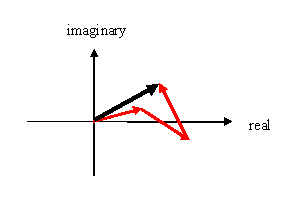We have to add every other bit of f(x) together, which will give some final complex vector, pointing off in some direction. F(0) will (in general) be a complex number.

Now what about the Fourier transform at other values θ?

To think this one through, imagine the mechanical device shown below: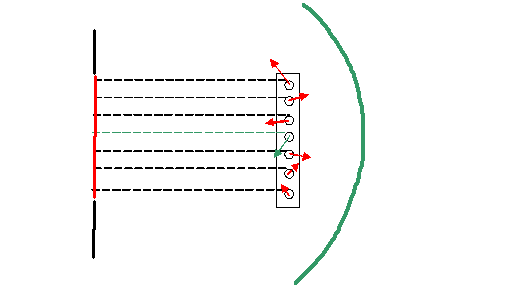In this diagram, rather than thinking about millions of threads (each coming from a tiny bit of f(x)), I’ve just drawn seven threads, as if f(x) was made up of seven slits (each of which is represented by a complex number). The threads are wrapped around little wheels, each of which has a circumference of exactly λ, the phase thread (electron wave) wavelength. Attached to the wheels are little wave values. To calculate the Fourier transform at an angle θ, we rotate the mechanical device around the green circle, like this: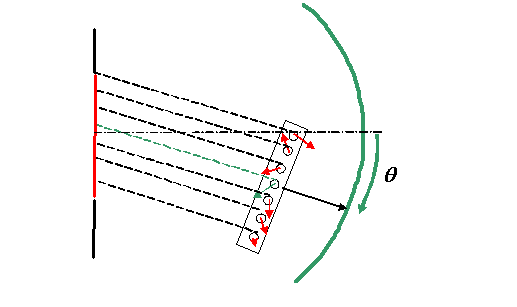Of course, some phase threads get shorter, others get longer, and, as they wrap around the wheels, their associated wave values cycle around in phase. (We assume the phase threads are kept taught). The Fourier transform of the wave represented by f(x) is just the addition of all these wave values (vectors) as a function of θ.

We’ve already said that at θ =0, this integral (the addition of the all the wave values) is just the complex integral of the original function. At some angle θ, we have to modify the phase of each bit of f(x) (i.e. the length of its associate phase thread, and the hence the phase angle of that particular bit of f(x)) before we do the integral.

How do we represent this mathematically?

Well, consider a bit of f(x) positioned at a distance x = D, that is f(D), where D is a constant. At an angle θ, the extra path length in the phase thread associated with this bit of f(x) is p = D sin θ. We’ve already said that we only interested in small values of θ, so we can say approximately that p = Dθ. The phase change in radians associated with this extra path length is just 2πDθ/ λ. So, the complex value of this phase thread is just f(D) changed in phase by 2π p/λ. We could write f(D) as the product of its modulus and phase, that is: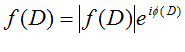where φ(D) is the phase of f(D). The extra phase change due to the extra length of phase at this particular value of θ is added to this value. Call the resultant complex wave value for this waved thread W(D), then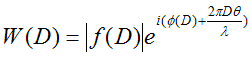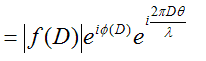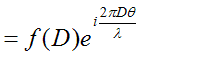Of course, the same argument applies for all D=x, so when we add up all the phase thread contributions, we get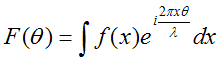which is a mathematical formulation of both the Fraunhofer diffraction amplitude and of the Fourier transform.

Remember: to form a Fourier transform, we plot as a function of angle θ the addition of all phase thread values, taking into account their differences in path length at a particular values of θ, where the phase threads start off at θ= 0 with the modulus and phase of the function we are Fourier transforming.

Of course, at θ=0, the exponential is unity, giving simply the integral of f(x), as we saw above.

Okay, well we’ve made a few approximations here, both from the point of view of the mathematics of a Fourier transform and the actual diffracted amplitude in a real scattering experiment, but the basic concept is correct.

From a mathematical point of view, it is most elegant to define the Fourier transform as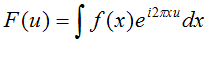Here, the coordinate of the Fourier transform is u and wavelength dependence (which is physical quantity, but not a relevant mathematical quantity) has been dropped. The beauty of this simpler definition is that the ‘back’ Fourier transform, that is the thing we do to F(u) to work out f(x), is just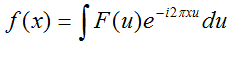The back transform is identical to the forward transform, except for a swapping of coordinates and the minus sign in the exponential. Physically, you can think of the back transform as reversing the direction of travel of the waves back from the Fraunhofer pattern to the object plane (harbour wall). Backward travelling waves require the corkscrew (i.e. the sign of the exponential) to be reversed.

In fact, physicists (and most electron microscopists) leave the λ in the definition. If we also remember that 2π/λ is often called k, then the Fourier transform is usually defined by physicists as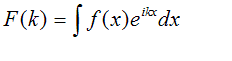At first, this may seem the simplest definition so far, because there are no constants in the exponential. However, if you work through the integral, the back transform now has to have a factor of 1/2π in front of it to keep the magnitude scaling constant. (This is because F(k) is ‘stretched out’ by a factor of 2π, and so the inverse integral is 2π times larger, because the area under the curve of F(k) is bigger by this factor). For two-dimensional transforms, this factor is squared (or raised to the power of N for N- dimensional transforms). Some people define both the forward and back transforms with a 1/(2π) N/2 in front of the integral to keep the apparent symmetry of the transforms. The forward transform is also sometimes defined with the minus sign in the exponential (in which case, the back transform has a positive exponential). All these variants you can find discussed in detail in the textbooks. The various definitions have historically developed in different fields of endeavour.